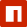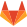# Calculator block

Use the calculator block to instantly perform calculations inside forms, using given answers. The block includes operations, scores, comparisons, formulas, functions, constants and lots more features to be able to do all kinds of calculations.

info

This is a headless block. It doesn't require a UI implementation in the runner. See the runner section for instructions on how to use the block in a runner.

## 📺 Preview​## 🧮 Supported operations​

OperationDescription
INPUTS
Static numberSupplies a static number to the calculator.
Recall valueSupplies the value of another question block (for example from a number block, rating block or scale block) to the calculator.
SubcalculationPerforms a (sub) calculation for multistep formulas and supplies the result to the calculator.
FUNCTIONS
Limiting
minReturns the input with the lowest number.
maxReturns the input with the highest number.
clampClamps (restricts) the input between the specified minimum and maximum value (learn more).
Floating point
truncRemoves decimals from a floating point number.
Exponentiation
powCalculates the base to the power of the given exponent (learn more).
expCalculates `e` to the power of the given exponent (learn more).
Trigonometry
sinh⁻¹Calculates the inverse hyperbolic sine (arcsine) of the given number (learn more).
cosh⁻¹Calculates the inverse hyperbolic cosine (arccosine) of the given number (learn more).
tanh⁻¹Calculates the inverse hyperbolic tangent (arctangent) of the given number (learn more).
Factorial
Miscellaneous
ageCalculates the age based on the input of a date block, for example a date of birth.
yearCalculates the year of a given date.
monthCalculates the month of a given date.
day of monthCalculates the day of the month of a given date.
day of weekCalculates the day of the week of a given date.
hourCalculates the hour of a given time.
minuteCalculates the minute of a given time.
secondCalculates the second of a given time.
millisecondCalculates the millisecond of a given time.
countCounts the number of selected options, for example from a checkboxes block, or a picture choice block (multiple selection).
scoreScores options of a block (for example a dropdown block, radio buttons block or a picture choice block).
COMPARATORS
Compare valueCompares the recalled value of another block and outputs a value based on the result of the comparison.
Compare current outcomeCompares the current outcome of the calculator and outputs a value based on the result of the comparison.
Compare numberCompares a static number and outputs a value based on the result of the comparison.
Compare date/timeCompares a date (and time) and outputs a value based on the result of the comparison.
Check selected optionChecks if a certain option is checked (for example from a multiple choice block) and outputs a value based on the result of the comparison.
TEXT FUNCTIONS
Character countCounts the number of characters in a text.
Word countCounts the number of words in a text.
Line countCounts the number of lines in a text.
Count occurrencesCounts the number of occurrences of a certain text or character.
Convert to numberConverts a text value to a number.
CONSTANTS
πSupplies the constant value of `π (pi ≈ 3.14159)` to the calculator (learn more).
eSupplies the constant value of `e (Euler's constant ≈ 2.71828)` to the calculator (learn more).
γSupplies the constant value of `γ (Euler–Mascheroni constant ≈ 0.57722)` to the calculator (learn more).
cSupplies the constant value of `c (speed of light = 299792458 m/s)` to the calculator (learn more).
Random valueSupplies a random value (0 to less than 1) to the calculator.
UNIX timeSupplies the current UNIX time (seconds since Unix Epoch) to the calculator.
YearSupplies the current year to the calculator.
MonthSupplies the current month (January = 1) to the calculator.
Day of monthSupplies the current day of month (1-31) to the calculator.
Day of weekSupplies the current day of week (Sunday = 0) to the calculator.
HourSupplies the current hour (0-23) to the calculator.
MinuteSupplies the current minute (0-59) to the calculator.
SecondSupplies the current second (0-59) to the calculator.
MillisecondSupplies the current millisecond (0-999) to the calculator.
TimezoneSupplies the current timezone (in milliseconds) to the calculator.

## 📦 Package contents​

### 🏗️ Builder part​

The builder part instructs the builder how to manage the block. It defines the configurable properties and settings for the block, which can then be managed with the visual builder. This package contains:

• Classes:
• `Calculator`: Calculator headless block;
• `Operation`: Operation item in the calculator collection;
• `CalculatorCondition`: Calculator condition block to verify a calculator outcome;
• TypeScript typings (should work out-of-the-box);
• Translations (located in the `./translations` folder of the package).

### 🏃 Runner part​

The runner part of this headless block contains all the code to use the block in a runner (the thing that runs the form). This package contains:

• Classes:
• `Calculator`: Base class for implementing the calculator block in a runner;
• `CalculatorCondition`: Runner part of the calculator condition block;
• TypeScript typings (should work out-of-the-box).

## 👩‍💻 Usage​

### ✨ Installation​

``npm install @tripetto/block-calculator``
Typescript support

This package contains type declarations and supports TypeScript out-of-the-box.

### 🏗️ Builder part​

#### ESM/ES5​

``import "@tripetto/block-calculator";// The code above imports the ES5 or ESM version based on your project configuration.// If you want to use the ES5 version, you can do an explicit import:import "@tripetto/block-calculator/es5";``

#### CDN​

You can also use the builder part directly in a browser using a CDN (see the builder HTML implementation for more information).

``<html>  <body>    <script src="https://unpkg.com/@tripetto/builder"></script>    <script src="https://unpkg.com/@tripetto/block-calculator"></script>    <script>      Tripetto.Builder.open();    </script>  </body></html>``

#### Translations​

The available translation for the block are located in the `translations` folder of the package.

tip

See the Loading a translation for a stock block guide to learn how to load the block translation into the builder.

### 🏃 Runner part​

Since this is a headless block, the runner part contains everything you need to use this block in a runner (the thing that runs the form). You only need to import the block somewhere in your runner project, and you are good to go:

``import "@tripetto/block-calculator/runner";// The code above imports the ES5 or ESM version based on your project configuration.// If you want to use the ES5 version, you can do an explicit import:import "@tripetto/block-calculator/runner/es5";``

## 🎭 Stock runners​

This block is included in the following stock runners:

tip

If you are integrating the builder together with one of the stock runners, you can use the builder block bundle that is included in the stock runner packages to load the builder part of all the blocks with a single import. See the Import block bundles guide for more information.

## 🚢 Distribution​

This block is distributed through npm:

## 📁 Source code​

This block is open-source and the code is on GitLab: